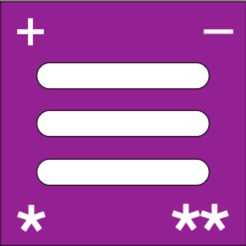## iPhone 屏幕快照

•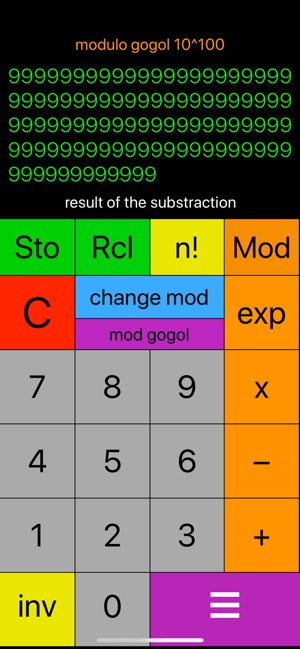•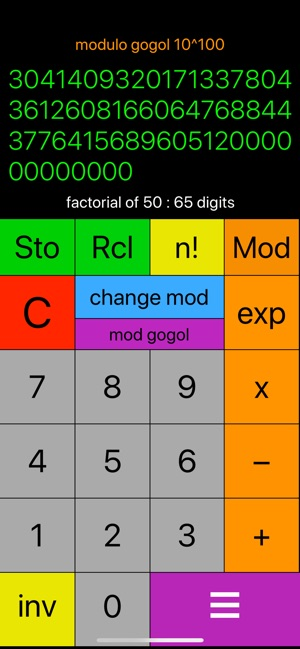•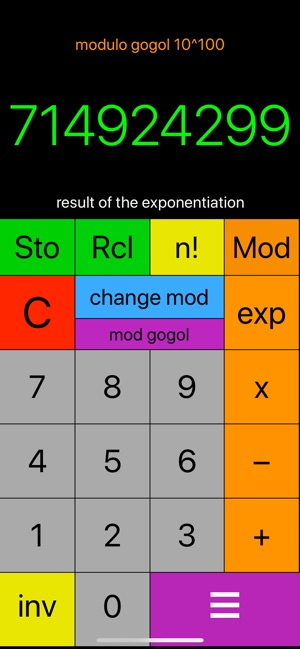•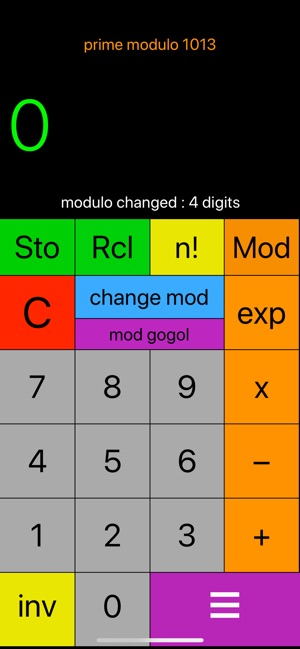•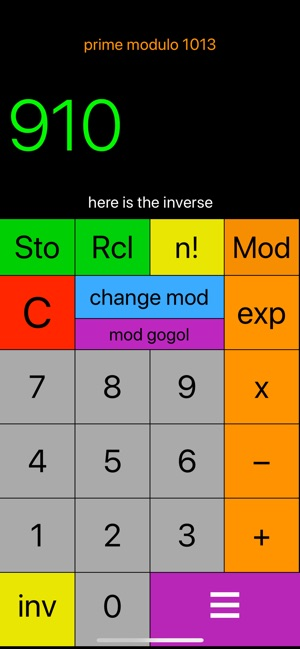•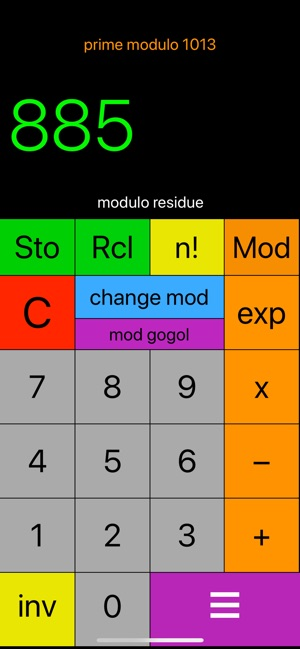••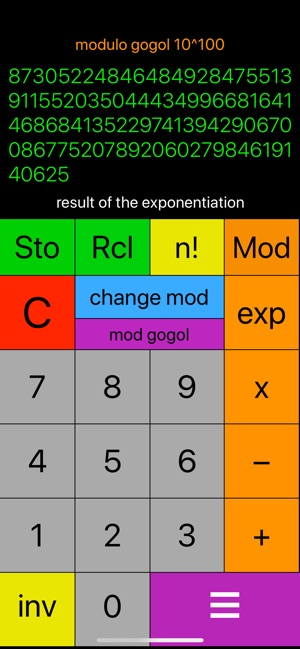## 简介

What are all the digits of the factorial of 69 and how many digits ?
What is the gogol number ?

How many integers of the form 10^n+1 are prime ? (like 101)

Want to know if a big integer is prime ? The change mod function indicates when the number is prime.

Want to know the divisors of an integer ? A prime divisor has no inverse modulo this integer.

This is a modulo calculator overpowering almost limitless! (only display is limited !)

With modulo gogol, calculus are exact until 100 digits !

The "n!" button calculates the factorial of an integer from 0 to 500. Factorial are limited to factorial of 500, but the calculator could calculate them to over 1000 ! (soon on iPad)

The "change mod" (change modulo) function indicates when the modulo is prime. There are no limit to the modulo except the display.

The "mod gogol" button set a modulo to 10^100 (gogol number).

You can stoke one number and recall it when you want.

The "Mod" operation indicates the residue modulo N.

The exp function allow modular exponentiation (as + - *)

The "INV" function calculates the inverse of an integer modulo N.

This app is intended for both mathematics teachers and/or their students, or if you are interested in the beauty of numbers.

Enjoy calcMod-ProX !

Les commentaires sont disponibles en français (réglages).

## 新内容

Fixed a bug linked to Boolean for RCL and aritmetic operations

## 信息

Alain Cardinaux

14.6 MB

Copyright
© 2018 Alain Cardinaux

¥6.00

## 支持

•